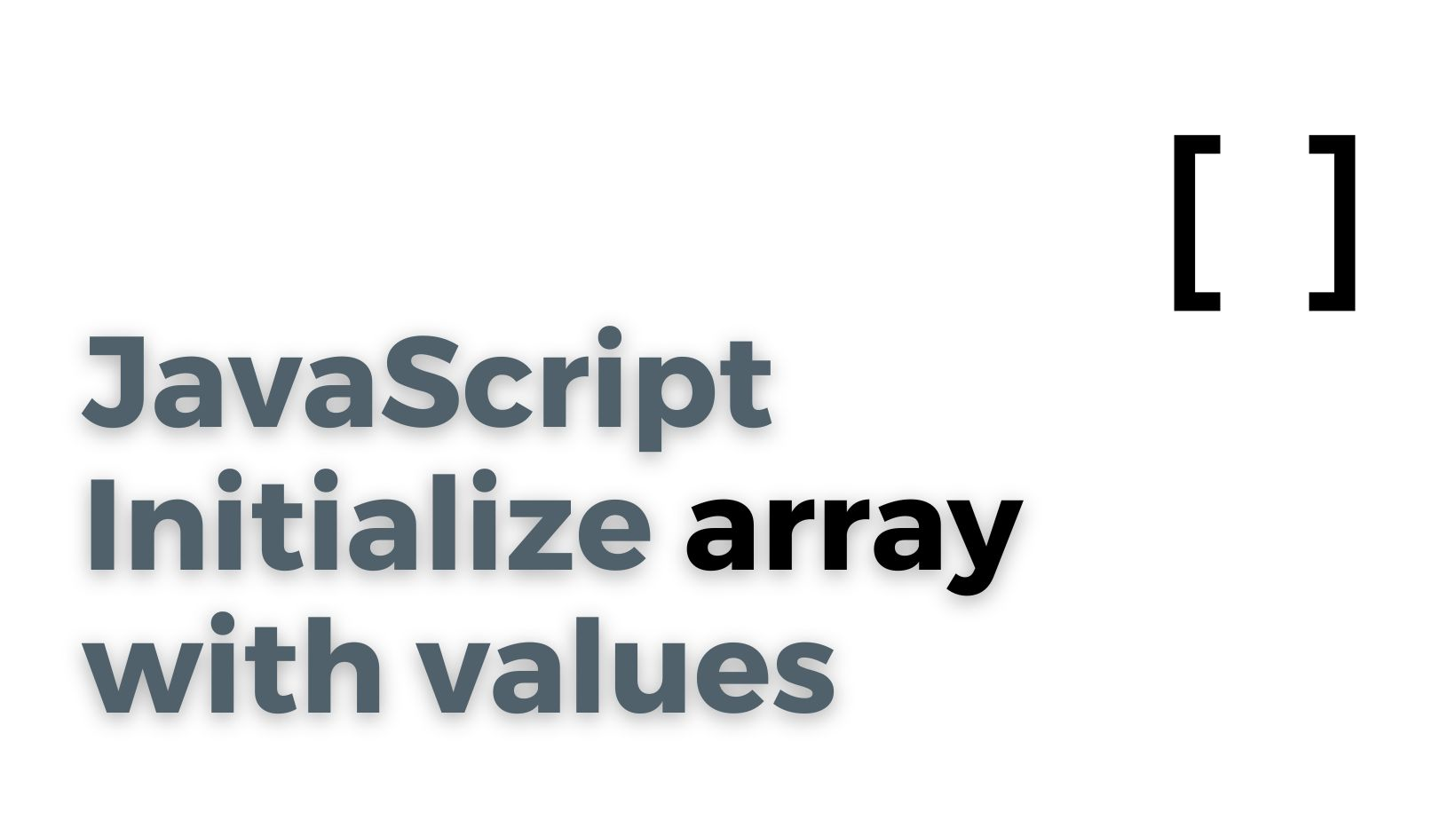# JavaScript - Initialize array with valuesIn JavaScript, an array is a data type that is used to store a collection of values. Arrays are a useful and versatile data structure that allow you to store and manipulate multiple values in a single variable.

An array in JavaScript is an object that contains a numbered list of values. Each value in the array is called an element, and each element has a corresponding numeric index that can be used to access it.

In addition to storing numeric values, arrays in JavaScript can also store other data types, such as strings, booleans, and even other arrays. This makes them a powerful tool for organizing and manipulating data in your code.

## #Initialize an array using Array constructor

To initialize an array in JavaScript with specific values, you can use the `Array` constructor and pass in the values you want to include in the array. For example, if you want to create an array that contains the numbers 1, 2, and 3, you can use the following code:

``````var myArray = new Array(1, 2, 3);
``````

## #Initialize an array using Square bracket notation

You can use the square bracket notation to create the array and specify the values at the same time, like this:

``````var myArray = [1, 2, 3];
``````

Once you have created the array, you can access its elements using the square bracket notation and the index of the element you want to access. For example, to access the first element in the `myArray` variable, you would use the following code:

``````console.log(myArray);  // Output: 1
``````

You can also use the square bracket notation to modify the values in the array. For example, if you want to change the value of the second element in `myArray` to 4, you can use the following code:

``````myArray = 4;
``````

After running this code, the `myArray` variable would contain the following elements: `[1, 4, 3]`.

## #Initialize an array using Array.of()

You can use the `Array.of()` method to create an array from a list of values, like this:

``````var myArray = Array.of(1, 2, 3);
``````

This creates an array that contains the same values as the `Array` constructor method shown above, but it uses a different syntax.

## #Initialize an array using Array.from()

Another way to initialize an array is to use the `Array.from()` method, which creates an array from an array-like object or iterable. For example, you could create an array from a string by using the `split()` method, like this:

``````var myString = "1,2,3";
var myArray = Array.from(myString.split(","));
``````

This creates an array that contains the same values as the examples above, but it uses the `Array.from()` method to do so.

## #Initialize an array using spread operator

Finally, you can also use the spread operator (`...`) to create an array from a list of values. This syntax is similar to the `Array.of()` method, but it uses the spread operator instead. For example:

``````var myArray = [...1, 2, 3];
``````

This creates an array that contains the same values as the other examples, but it uses the spread operator to do so.

## Related articlesWritten by author: Ruslan Osipov